# Spectral asymptotics of self-adjoint fourth order boundary value problems with eigenvalue parameter dependent boundary conditions

## Abstract

A regular fourth order differential equation with λ-dependent boundary conditions is considered. For four distinct cases with exactly one λ-independent boundary condition, the asymptotic eigenvalue distribution is presented.

MSC:34L20, 34B07, 34B08, 34B09.

## 1 Introduction

Sturm-Liouville problems have attracted extensive attention due to their intrinsic mathematical challenges and their applications in physics and engineering. However, apart from classical Sturm-Liouville problems, also higher order ordinary linear differential equations occur in applications, with or without the eigenvalue parameter in the boundary conditions. Such problems are realized as operator polynomials, also called operator pencils. Some recent developments of higher order differential operators whose boundary conditions depend on the eigenvalue parameter, including spectral asymptotics and basis properties, have been investigated in . General characterizations of self-adjoint boundary conditions have been presented in [5, 6] for singular and (quasi-)regular problems. In all these cases, the minimal operator associated with an n th order differential equation must be symmetric, see [7, 8] for necessary and sufficient conditions. A more general discussion on the spectra of fourth order differential operators can be found in [9, 10].

The generalized Regge problem is realized by a second order differential operator which depends quadratically on the eigenvalue parameter and which has eigenvalue parameter dependent boundary conditions, see . The particular feature of this problem is that the coefficient operators of this pencil are self-adjoint, and it is shown in  that this gives some a priori knowledge about the location of the spectrum. In  this approach has been extended to a fourth order differential equation describing small transversal vibrations of a homogeneous beam compressed or stretched by a force g. Separation of variables leads to a fourth order boundary problem with eigenvalue parameter dependent boundary conditions, where the differential equation

${y}^{\left(4\right)}-{\left(g{y}^{\prime }\right)}^{\prime }={\lambda }^{2}y$

depends quadratically on the eigenvalue parameter. This problem is represented by a quadratic operator pencil, in a suitably chosen Hilbert space, whose coefficient operators are self-adjoint. In  we have investigated a class of boundary conditions for which necessary and sufficient conditions were obtained such that the associated operator pencil consists of self-adjoint operators, while in  we have continued the work of  in the direction of  to derive eigenvalue asymptotics associated with boundary conditions which lead to self-adjoint operator representations. We have considered the particular case of boundary conditions which do not depend on the eigenvalue parameter at the left endpoint and depend on the eigenvalue parameter at the right endpoint.

In this paper, we extend the work of  to a class of boundary conditions where exactly one of the left endpoint boundary conditions does not depend on the eigenvalue parameter, while the remaining boundary conditions depend on the eigenvalue parameter.

We define the operator pencil in Section 2 and we discuss which boundary conditions are considered. In Section 3, the eigenvalue asymptotics for the case $g=0$ are derived. In Section 4, it is shown that the boundary value problems under consideration are Birkhoff regular, which implies that the eigenvalues for general g are small perturbations of the eigenvalues for $g=0$. Hence, in Section 5, the first four terms of the eigenvalue asymptotics are found and are compared to those obtained in .

## 2 The quadratic operator pencil L

On the interval $\left[0,a\right]$, we consider the boundary value problem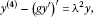(2.1)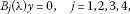(2.2)

where $a>0$, $g\in {C}^{1}\left[0,a\right]$ is a real valued function and (2.2) are separated boundary conditions where the ${B}_{j}\left(\lambda \right)$ are constant or depend on λ linearly. The boundary conditions (2.2) are taken at the endpoint 0 for $j=1,2$ and at the endpoint a for $j=3,4$. Further, we assume for simplicity that either ${B}_{j}\left(\lambda \right)y={y}^{\left[{p}_{j}\right]}\left({a}_{j}\right)+i{\epsilon }_{j}\alpha \lambda {y}^{\left[{q}_{j}\right]}\left({a}_{j}\right)$ or ${B}_{j}\left(\lambda \right)y={y}^{\left[{p}_{j}\right]}\left({a}_{j}\right)$, where ${a}_{j}=0$ for $j=1,2$, ${a}_{j}=a$ for $j=3,4$, $\alpha >0$ and ${\epsilon }_{j}\in \left\{-1,1\right\}$. We recall that the quasi-derivatives associated with (2.1) are given by

${y}^{\left[0\right]}=y,\phantom{\rule{2em}{0ex}}{y}^{\left[1\right]}={y}^{\prime },\phantom{\rule{2em}{0ex}}{y}^{\left[2\right]}={y}^{″},\phantom{\rule{2em}{0ex}}{y}^{\left[3\right]}={y}^{\left(3\right)}-g{y}^{\prime },\phantom{\rule{2em}{0ex}}{y}^{\left[4\right]}={y}^{\left(4\right)}-{\left(g{y}^{\prime }\right)}^{\prime },$

see [, p.26].

Define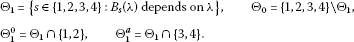Assumption 2.1 The numbers ${p}_{1}$, ${p}_{2}$, ${q}_{j}$ for $j\in {\mathrm{\Theta }}_{1}^{0}$ are distinct as well as the numbers ${p}_{3}$, ${p}_{4}$, ${q}_{j}$ for $j\in {\mathrm{\Theta }}_{1}^{a}$.

We denote by U the collection of the boundary conditions (2.2) and define the following operators related to U:

${U}_{0}y={\left({y}^{\left[{p}_{j}\right]}\left({a}_{j}\right)\right)}_{j\in {\mathrm{\Theta }}_{1}}\phantom{\rule{1em}{0ex}}\text{and}\phantom{\rule{1em}{0ex}}{U}_{1}y={\left({\epsilon }_{j}{y}^{\left[{q}_{j}\right]}\left({a}_{j}\right)\right)}_{j\in {\mathrm{\Theta }}_{1}},\phantom{\rule{1em}{0ex}}y\in {W}_{4}^{2}\left(0,a\right).$
(2.3)

We put $k=|{\mathrm{\Theta }}_{1}|$ and consider the linear operators $A\left(U\right)$, K and M in the space ${L}_{2}\left(0,a\right)\oplus {\mathbb{C}}^{k}$ with domains

given by

It is easy to check that $K\ge 0$, $M\ge 0$, $M+K=I$ and $M{|}_{\mathcal{D}\left(A\left(U\right)\right)}>0$. We associate a quadratic operator pencil

$L\left(\lambda ,\alpha \right)={\lambda }^{2}M-i\alpha \lambda K-A\left(U\right),\phantom{\rule{1em}{0ex}}\lambda \in \mathbb{C}$
(2.4)

in the space ${L}_{2}\left(0,a\right)\oplus {\mathbb{C}}^{k}$ with the problem (2.1), (2.2).

The conditions under which the differential operator $A\left(U\right)$ is self-adjoint are given in

Theorem 2.2 (, Theorem 1.2)

Denote by ${P}_{0}$ the set of p in ${y}^{\left[p\right]}\left(0\right)=0$ for the λ-independent boundary conditions and by ${P}_{a}$ the corresponding set for ${y}^{\left[p\right]}\left(a\right)=0$. Then the differential operator $A\left(U\right)$ associated with this boundary value problem is self-adjoint if and only if $p+q=3$ for all boundary conditions of the form ${y}^{\left[p\right]}\left({a}_{j}\right)+i\alpha {\epsilon }_{j}\lambda {y}^{\left[q\right]}\left({a}_{j}\right)=0$ and ${\epsilon }_{j}=1$ if q is even in case ${a}_{j}=0$ or odd in case ${a}_{j}=a$, ${\epsilon }_{j}=-1$ otherwise, $\left\{0,3\right\}\not\subset {P}_{0}$, $\left\{1,2\right\}\not\subset {P}_{0}$, $\left\{0,3\right\}\not\subset {P}_{a}$ and $\left\{1,2\right\}\not\subset {P}_{a}$.

Proposition 2.3 The operator pencil $L\left(\cdot ,\alpha \right)$ is a Fredholm valued operator function with index 0. The spectrum of the Fredholm operator $L\left(\cdot ,\alpha \right)$ consists of discrete eigenvalues of finite multiplicities, and all eigenvalues of $L\left(\cdot ,\alpha \right)$, $\alpha \ge 0$, lie in the closed upper half-plane and on the imaginary axis and are symmetric with respect to the imaginary axis.

Proof As in [, Section 3], we can argue that for all $\lambda \in \mathbb{C}$, $L\left(\lambda ,\alpha \right)$ is a relatively compact perturbation of $L\left(0,0\right)$, where $L\left(0,0\right)$ is well known to be a Fredholm operator. The statement on the location of the spectrum now follows as in [, Lemma 3.1]. □

We now consider the particular cases that exactly one of the boundary conditions at 0 depends on λ, whereas both boundary conditions at a depend on λ. Therefore, taking Assumption 2.1 and Theorem 2.2 into account, we have the four boundary conditions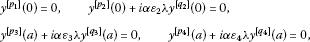where $0\le {p}_{1}\le 3$, $0\le {p}_{2}\le 3$, $0\le {q}_{2}\le 3$, ${p}_{2}+{q}_{2}=3$, and ${p}_{1}\notin \left\{{q}_{2},{p}_{2}\right\}$, while $\left\{{p}_{3},{q}_{3}\right\}=\left\{1,2\right\}$ and $\left\{{p}_{4},{q}_{4}\right\}=\left\{0,3\right\}$. Thus, we have 8 and 4 possible sets of boundary conditions at the endpoint 0 and a, respectively. Whence there are 32 different sets of boundary conditions. Recall that the parameter λ emanates from derivatives with respect to the time variable in the original partial differential equation, and it is reasonable that the highest space derivative occurs in the term without time derivative. Thus, the most relevant boundary conditions would have ${q}_{2}<{p}_{2}$, ${q}_{3}<{p}_{3}$ and ${q}_{4}<{p}_{4}$. This leaves us with four different cases for the boundary conditions ${B}_{j}\left(\lambda \right)y=0$.

These four cases are uniquely determined by the value of ${p}_{1}$, so that we will consider

Case 1: ${p}_{1}=3$; Case 2: ${p}_{1}=0$; Case 3: ${p}_{1}=1$; Case 4: ${p}_{1}=2$.

The corresponding boundary operators are then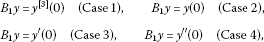(2.5)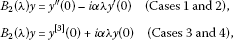(2.6)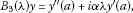(2.7)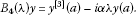(2.8)

## 3 Asymptotics of eigenvalues for $g=0$

In this section, we consider the boundary value problem (2.1), (2.2) with $g=0$. We count all eigenvalues with their proper multiplicities and develop a formula for the asymptotic distribution of the eigenvalues, which is used to obtain the corresponding formula for general g. Observe that for $g=0$, the quasi-derivatives ${y}^{\left[j\right]}$ coincide with the standard derivatives ${y}^{\left(j\right)}$. We take the canonical fundamental system ${y}_{j}\left(\cdot ,\lambda \right)$, $j=1,\dots ,4$, of (2.1) with ${y}_{j}^{\left(m\right)}\left(0\right)={\delta }_{j,m+1}$ if $j\ge 2$ for $m=0,\dots ,3$. It is well known that the functions ${y}_{j}\left(\cdot ,\lambda \right)$ are analytic on with respect to λ. Putting

$M\left(\lambda \right)={\left({B}_{i}\left(\lambda \right){y}_{j}\left(\cdot ,\lambda \right)\right)}_{i,j=1}^{4},$

the eigenvalues of the boundary value problem (2.1), (2.2) are the eigenvalues of the analytic matrix function M, where the corresponding geometric and algebraic multiplicities coincide, see [, Theorem 6.3.2].

Setting $\lambda ={\mu }^{2}$ and

$y\left(x,\mu \right)=\frac{1}{2{\mu }^{3}}sinh\left(\mu x\right)-\frac{1}{2{\mu }^{3}}sin\left(\mu x\right)$

it is easy to see that

${y}_{j}\left(x,\lambda \right)={y}^{\left(4-j\right)}\left(x,\mu \right),\phantom{\rule{1em}{0ex}}j=1,\dots ,4.$

The second row of $M\left(\lambda \right)$ has exactly two non-zero entries (for $\lambda \ne 0$), and these non-zero entries are:

In Cases 1 and 2, ${B}_{2}\left(\lambda \right){y}_{2}\left(\cdot ,\lambda \right)=-i\alpha {\mu }^{2}$ and ${B}_{2}\left(\lambda \right){y}_{3}\left(\cdot ,\lambda \right)=1$;

In Cases 3 and 4, ${B}_{2}\left(\lambda \right){y}_{1}\left(\cdot ,\lambda \right)=i\alpha {\mu }^{2}$ and ${B}_{2}\left(\lambda \right){y}_{4}\left(\cdot ,\lambda \right)=1$.

Since the first row of $M\left(\lambda \right)$ has exactly one entry 1 and all other entries zero, an expansion of $M\left(\lambda \right)$ with respect to the second row shows that $detM\left(\lambda \right)=±\varphi \left(\mu \right)$, where

$\begin{array}{rcl}\varphi \left(\mu \right)& =& i\alpha {\mu }^{2}det\left(\begin{array}{cc}{B}_{3}\left({\mu }^{2}\right){y}_{\sigma \left(1\right)}\left(\cdot ,\mu \right)& {B}_{3}\left({\mu }^{2}\right){y}_{\sigma \left(2\right)}\left(\cdot ,\mu \right)\\ {B}_{4}\left({\mu }^{2}\right){y}_{\sigma \left(1\right)}\left(\cdot ,\mu \right)& {B}_{4}\left({\mu }^{2}\right){y}_{\sigma \left(2\right)}\left(\cdot ,\mu \right)\end{array}\right)\\ +det\left(\begin{array}{cc}{B}_{3}\left({\mu }^{2}\right){y}_{\sigma \left(3\right)}\left(\cdot ,\mu \right)& {B}_{3}\left({\mu }^{2}\right){y}_{\sigma \left(4\right)}\left(\cdot ,\mu \right)\\ {B}_{4}\left({\mu }^{2}\right){y}_{\sigma \left(3\right)}\left(\cdot ,\mu \right)& {B}_{4}\left({\mu }^{2}\right){y}_{\sigma \left(4\right)}\left(\cdot ,\mu \right)\end{array}\right),\end{array}$

with

$\left(\sigma \left(1\right),\sigma \left(2\right),\sigma \left(3\right),\sigma \left(4\right)\right)=\left\{\begin{array}{cc}\left(1,3,1,2\right)\hfill & \text{in Case 1},\hfill \\ \left(3,4,2,4\right)\hfill & \text{in Case 2},\hfill \\ \left(3,4,1,3\right)\hfill & \text{in Case 3},\hfill \\ \left(2,4,1,2\right)\hfill & \text{in Case 4}.\hfill \end{array}$

In view of (2.7), (2.8) this gives

$\begin{array}{rcl}\varphi \left(\mu \right)& =& i\alpha {\mu }^{2}\left[\left({y}_{\sigma \left(1\right)}^{″}\left(a,\mu \right)+i\alpha {\mu }^{2}{y}_{\sigma \left(1\right)}^{\prime }\left(a,\mu \right)\right)\left({y}_{\sigma \left(2\right)}^{\left(3\right)}\left(a,\mu \right)-i\alpha {\mu }^{2}{y}_{\sigma \left(2\right)}\left(a,\mu \right)\right)\\ -\left({y}_{\sigma \left(2\right)}^{″}\left(a,\mu \right)+i\alpha {\mu }^{2}{y}_{\sigma \left(2\right)}^{\prime }\left(a,\mu \right)\right)\left({y}_{\sigma \left(1\right)}^{\left(3\right)}\left(a,\mu \right)-i\alpha {\mu }^{2}{y}_{\sigma \left(1\right)}\left(a,\mu \right)\right)\right]\\ +\left({y}_{\sigma \left(3\right)}^{″}\left(a,\mu \right)+i\alpha {\mu }^{2}{y}_{\sigma \left(3\right)}^{\prime }\left(a,\mu \right)\right)\left({y}_{\sigma \left(4\right)}^{\left(3\right)}\left(a,\mu \right)-i\alpha {\mu }^{2}{y}_{\sigma \left(4\right)}\left(a,\mu \right)\right)\\ -\left({y}_{\sigma \left(4\right)}^{″}\left(a,\mu \right)+i\alpha {\mu }^{2}{y}_{\sigma \left(4\right)}^{\prime }\left(a,\mu \right)\right)\left({y}_{\sigma \left(3\right)}^{\left(3\right)}\left(a,\mu \right)-i\alpha {\mu }^{2}{y}_{\sigma \left(3\right)}\left(a,\mu \right)\right)\\ =& i\alpha {\mu }^{2}\left[i\alpha {\mu }^{2}\left\{{y}_{\sigma \left(1\right)}^{\prime }\left(a,\mu \right){y}_{\sigma \left(2\right)}^{\left(3\right)}\left(a,\mu \right)-{y}_{\sigma \left(2\right)}^{\prime }\left(a,\mu \right){y}_{\sigma \left(1\right)}^{\left(3\right)}\left(a,\mu \right)\\ +{y}_{\sigma \left(2\right)}^{″}\left(a,\mu \right){y}_{\sigma \left(1\right)}\left(a,\mu \right)-{y}_{\sigma \left(1\right)}^{″}\left(a,\mu \right){y}_{\sigma \left(2\right)}\left(a,\mu \right)\right\}\\ +{\alpha }^{2}{\mu }^{4}\left\{{y}_{\sigma \left(1\right)}^{\prime }\left(a,\mu \right){y}_{\sigma \left(2\right)}\left(a,\mu \right)-{y}_{\sigma \left(2\right)}^{\prime }\left(a,\mu \right){y}_{\sigma \left(1\right)}\left(a,\mu \right)\right\}\\ +{y}_{\sigma \left(1\right)}^{″}\left(a,\mu \right){y}_{\sigma \left(2\right)}^{\left(3\right)}\left(a,\mu \right)-{y}_{\sigma \left(2\right)}^{″}\left(a,\mu \right){y}_{\sigma \left(1\right)}^{\left(3\right)}\left(a,\mu \right)\right]\\ +i\alpha {\mu }^{2}\left[{y}_{\sigma \left(3\right)}^{\prime }\left(a,\mu \right){y}_{\sigma \left(4\right)}^{\left(3\right)}\left(a,\mu \right)-{y}_{\sigma \left(4\right)}^{\prime }\left(a,\mu \right){y}_{\sigma \left(3\right)}^{\left(3\right)}\left(a,\mu \right)\\ +{y}_{\sigma \left(4\right)}^{″}\left(a,\mu \right){y}_{\sigma \left(3\right)}\left(a,\mu \right)-{y}_{\sigma \left(3\right)}^{″}\left(a,\mu \right){y}_{\sigma \left(4\right)}\left(a,\mu \right)\right]\\ +{\alpha }^{2}{\mu }^{4}\left({y}_{\sigma \left(3\right)}^{\prime }\left(a,\mu \right){y}_{\sigma \left(4\right)}\left(a,\mu \right)-{y}_{\sigma \left(4\right)}^{\prime }\left(a,\mu \right){y}_{\sigma \left(3\right)}\left(a,\mu \right)\right)\\ +{y}_{\sigma \left(3\right)}^{″}\left(a,\mu \right){y}_{\sigma \left(4\right)}^{\left(3\right)}\left(a,\mu \right)-{y}_{\sigma \left(4\right)}^{″}\left(a,\mu \right){y}_{\sigma \left(3\right)}^{\left(3\right)}\left(a,\mu \right).\end{array}$

Each of the summands in ϕ is a product of a power in μ and a product of two sums of a trigonometric and a hyperbolic functions. The terms with the highest μ-powers in $\varphi \left(\mu \right)$ are non-zero constant multiples of

${\varphi }_{0}\left(\mu \right)=\left\{\begin{array}{cc}2{\mu }^{4}\left({y}_{\sigma \left(1\right)}^{\prime }\left(a,\mu \right){y}_{\sigma \left(2\right)}^{\left(3\right)}\left(a,\mu \right)-{y}_{\sigma \left(2\right)}^{\prime }\left(a,\mu \right){y}_{\sigma \left(1\right)}^{\left(3\right)}\left(a,\mu \right)\right)\hfill & \text{in Cases 1, 2},\hfill \\ 2{\mu }^{2}\left({y}_{\sigma \left(3\right)}^{\prime }\left(a,\mu \right){y}_{\sigma \left(4\right)}^{\left(3\right)}\left(a,\mu \right)-{y}_{\sigma \left(4\right)}^{\prime }\left(a,\mu \right){y}_{\sigma \left(3\right)}^{\left(3\right)}\left(a,\mu \right)\right)\hfill & \text{in Cases 3, 4}.\hfill \end{array}$

For the above four cases, we obtain: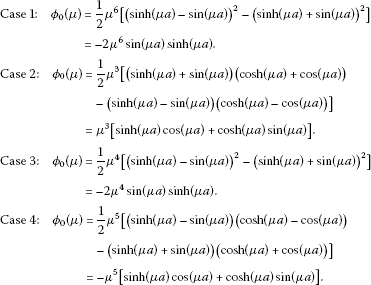We next give the asymptotic distributions of the zeros of ${\varphi }_{0}\left(\mu \right)$ with proper counting.

Lemma 3.1 Case 1: ${\varphi }_{0}$ has a zero of multiplicity 8 at 0, simple zeros at

${\stackrel{˜}{\mu }}_{k}=\left(k-2\right)\frac{\pi }{a},\phantom{\rule{1em}{0ex}}k=3,4,\dots ,$

simple zeros at $-{\stackrel{˜}{\mu }}_{k}$, ${\stackrel{˜}{\mu }}_{-k}=i{\stackrel{˜}{\mu }}_{k}$ and $-i{\stackrel{˜}{\mu }}_{k}$ for $k=3,4,\dots$, and no other zeros.

Case 2: ${\varphi }_{0}$ has a zero of multiplicity 4 at 0, exactly one simple zero ${\stackrel{˜}{\mu }}_{k}$ in each interval $\left(\left(k-\frac{1}{2}\right)\frac{\pi }{a},\left(k+\frac{1}{2}\right)\frac{\pi }{a}\right)$ for positive integers k with asymptotics

${\stackrel{˜}{\mu }}_{k}=\left(4k-5\right)\frac{\pi }{4a}+o\left(1\right),\phantom{\rule{1em}{0ex}}k=2,3,\dots ,$

simple zeros at $-{\stackrel{˜}{\mu }}_{k}$, ${\stackrel{˜}{\mu }}_{-k}=i{\stackrel{˜}{\mu }}_{k}$ and $-i{\stackrel{˜}{\mu }}_{k}$ for $k=2,3,\dots$ , and no other zeros.

Case 3: ${\varphi }_{0}$ has a zero of multiplicity 6 at 0, simple zeros at

${\stackrel{˜}{\mu }}_{k}=\left(k-1\right)\frac{\pi }{a},\phantom{\rule{1em}{0ex}}k=2,3,\dots ,$

simple zeros at $-{\stackrel{˜}{\mu }}_{k}$, ${\stackrel{˜}{\mu }}_{-k}=i{\stackrel{˜}{\mu }}_{k}$ and $-i{\stackrel{˜}{\mu }}_{k}$ for $k=2,3,\dots$, and no other zeros.

Case 4: ${\varphi }_{0}$ has a zero of multiplicity 6 at 0, exactly one simple zero ${\stackrel{˜}{\mu }}_{k}$ in each interval $\left(\left(k-\frac{1}{2}\right)\frac{\pi }{a},\left(k+\frac{1}{2}\right)\frac{\pi }{a}\right)$ for positive integers k with asymptotics

${\stackrel{˜}{\mu }}_{k}=\left(4k-5\right)\frac{\pi }{4a}+o\left(1\right),\phantom{\rule{1em}{0ex}}k=2,3,\dots ,$

simple zeros at $-{\stackrel{˜}{\mu }}_{k}$, ${\stackrel{˜}{\mu }}_{-k}=i{\stackrel{˜}{\mu }}_{k}$ and $-i{\stackrel{˜}{\mu }}_{k}$ for $k=2,3,\dots$, and no other zeros.

Proof The result is obvious in Cases 1 and 3. Cases 2 and 4 only differ in the factor with the power of μ, and the multiplicity of the corresponding zero of ${\varphi }_{0}$ at 0 is easy to verify. The choice of the indexing for the non-zero zeros of ${\varphi }_{0}$ in each case will become apparent later.

It, therefore, remains to describe the behavior of the non-zero zeros of ${\varphi }_{0}$ in Case 2. First, we are going to find the zeros of ${\varphi }_{0}$ on the positive real axis. One can observe that for $\mu \ne 0$, ${\varphi }_{0}\left(\mu \right)=0$ implies $cosh\left(\mu a\right)\ne 0$ and $cos\left(\mu a\right)\ne 0$, whence the positive zeros of ${\varphi }_{0}$ are those $\mu >0$ for which $tan\left(\mu a\right)+tanh\left(\mu a\right)=0$. Since ${tan}^{\prime }\left(\mu a\right)\ge 1$ and ${tanh}^{\prime }\left(\mu a\right)>0$ for all $x\in \mathbb{R}$ where the functions are defined, the function $\mu ↦tan\left(\mu a\right)+tanh\left(\mu a\right)$ is increasing with a positive derivative on each interval $\left(\left(k-\frac{1}{2}\right)\frac{\pi }{a},\left(k+\frac{1}{2}\right)\frac{\pi }{a}\right)$, $k\in \mathbb{Z}$. On each of these intervals, the function moves from −∞ to ∞, thus we have exactly one simple zero ${\stackrel{˜}{\mu }}_{k}$ of $tan\left(\mu a\right)+tanh\left(\mu a\right)$ in each interval $\left(\left(k-\frac{1}{2}\right)\frac{\pi }{a},\left(k+\frac{1}{2}\right)\frac{\pi }{a}\right)$, where k is a positive integer, and no zero in $\left(0,\frac{\pi }{2a}\right)$. Since $tanh\left(\mu a\right)\to 1$ as $\mu \to \mathrm{\infty }$, we have

${\stackrel{˜}{\mu }}_{k}=\left(4k-5\right)\frac{\pi }{4a}+o\left(1\right),\phantom{\rule{1em}{0ex}}k=1,2,\dots .$

The location of the zeros on the other three half-axes follows by repeated application of ${\varphi }_{0}\left(i\mu \right)=-{\varphi }_{0}\left(\mu \right)$.

The proof will be complete if we show that all zeros of ${\varphi }_{0}$ lie on the real or the imaginary axis. To this end, we observe that the product-to-sum formula for trigonometric functions gives

$\begin{array}{rcl}{\varphi }_{0}\left(\mu \right)& =& {\mu }^{3}\left[cosh\left(\mu a\right)sin\left(\mu a\right)+sinh\left(\mu a\right)cos\left(\mu a\right)\right]\\ =& \frac{1}{2}{\mu }^{3}\left[sin\left(\left(1+i\right)\mu a\right)+sin\left(\left(1-i\right)\mu a\right)\\ -isin\left(\left(1+i\right)\mu a\right)+isin\left(\left(1-i\right)\mu a\right)\right]\\ =& \frac{1}{2}{\mu }^{3}\left[\left(1-i\right)sin\left(\left(1+i\right)\mu a\right)+\left(1+i\right)sin\left(\left(1-i\right)\mu a\right)\right].\end{array}$
(3.1)

Putting $\left(1+i\right)\mu a=x+iy$, $x,y\in \mathbb{R}$, it follows for $\mu \ne 0$ that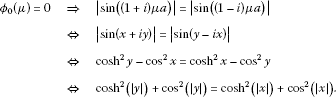(3.2)

Since ${cosh}^{2}x+{cos}^{2}x=\frac{1}{2}cosh\left(2x\right)+\frac{1}{2}cos\left(2x\right)+1$ has a positive derivative on $\left(0,\mathrm{\infty }\right)$, this function is strictly increasing, and ${\varphi }_{0}\left(\mu \right)=0$ therefore, implies by (3.2) that $|y|=|x|$ and thus $y=±x$. Then

$\mu =\frac{x+iy}{\left(1+i\right)a}=\frac{1±i}{1+i}\frac{x}{a}$

is either real or pure imaginary. □

Proposition 3.2 For $g=0$, there exists a positive integer ${k}_{0}$ such that the eigenvalues ${\stackrel{ˆ}{\lambda }}_{k}$, counted with multiplicity, of the problem (2.1), (2.5)-(2.8), where $k\in \mathbb{Z}\setminus \left\{0\right\}$ in Cases 1 and  2 and $k\in \mathbb{Z}$ in Cases 3 and 4, can be enumerated in such a way that the eigenvalues ${\stackrel{ˆ}{\lambda }}_{k}$ are pure imaginary for $|k|<{k}_{0}$, and ${\stackrel{ˆ}{\lambda }}_{-k}=-\overline{{\stackrel{ˆ}{\lambda }}_{k}}$ for $k\ge {k}_{0}$. For $k>0$, we can write ${\stackrel{ˆ}{\lambda }}_{k}={\stackrel{ˆ}{\mu }}_{k}^{2}$, where the ${\stackrel{ˆ}{\mu }}_{k}$ have the following asymptotic representation as $k\to \mathrm{\infty }$: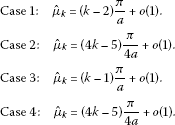In particular, the number of pure imaginary eigenvalues is even in Cases 1 and 2 and odd in Cases 3 and 4.

Proof Case 4: A straightforward calculation gives

$\begin{array}{rcl}\varphi \left(\mu \right)& =& -\frac{1}{2}i\left(2\alpha +{\alpha }^{3}\right){\mu }^{3}\left[cosh\left(\mu a\right)sin\left(\mu a\right)-sinh\left(\mu a\right)cos\left(\mu a\right)\right]\\ -\frac{1}{2}i\alpha {\mu }^{5}\left[sinh\left(\mu a\right)cos\left(\mu a\right)+cosh\left(\mu a\right)sin\left(\mu a\right)\right]\\ -\frac{1}{2}{\alpha }^{2}{\mu }^{4}\left[3cosh\left(\mu a\right)cos\left(\mu a\right)+1\right]-\frac{1}{2}{\mu }^{4}\left[cosh\left(\mu a\right)cos\left(\mu a\right)-1\right]\\ -{\alpha }^{2}{\mu }^{2}sin\left(\mu a\right)sinh\left(\mu a\right).\end{array}$
(3.3)

Up to the constant factor $\frac{1}{2}i\alpha$, the second term equals ${\varphi }_{0}\left(\mu \right)$. It follows that for μ outside the zeros of ${\varphi }_{0}$, $cos\left(\cdot a\right)$ and $cosh\left(\cdot a\right)$, we have

$\begin{array}{rcl}{\varphi }_{1}\left(\mu \right)=\frac{2\varphi \left(\mu \right)-i\alpha {\varphi }_{0}\left(\mu \right)}{i\alpha {\varphi }_{0}\left(\mu \right)}& =& \frac{{\alpha }^{2}-1}{i\alpha \mu }\frac{1}{cosh\left(\mu a\right)cos\left(\mu a\right)}\frac{1}{tan\left(\mu a\right)+tanh\left(\mu a\right)}\\ +\frac{3{\alpha }^{2}+1}{i\alpha \mu }\frac{1}{tan\left(\mu a\right)+tanh\left(\mu a\right)}+\frac{2\alpha }{i{\mu }^{3}}\frac{tan\left(\mu a\right)tanh\left(\mu a\right)}{tan\left(\mu a\right)+tanh\left(\mu a\right)}\\ +\frac{\left(2+{\alpha }^{2}\right)}{{\mu }^{2}}\left[1-2\frac{tanh\left(\mu a\right)}{tan\left(\mu a\right)+tanh\left(\mu a\right)}\right].\end{array}$
(3.4)

Fix $\epsilon \in \left(0,\frac{\pi }{4a}\right)$ and for $k=2,3,\dots$ let ${R}_{k,\epsilon }$ be the squares determined by the vertices $\left(4k-5\right)\frac{\pi }{4a}±\epsilon ±i\epsilon$, $k\in \mathbb{Z}$. These squares do not intersect due to $\epsilon <\frac{\pi }{2a}$. Since $tanz=-1$ if and only if $z=j\pi -\frac{\pi }{4}$ and $j\in \mathbb{Z}$, it follows from the periodicity of tan that the number

${C}_{1}\left(\epsilon \right)=2min\left\{|tan\left(\mu a\right)+1|:\mu \in {R}_{k,\epsilon }\right\}$

is positive and independent of ε. Since $tanh\left(\mu a\right)\to 1$ uniformly in the strip $\left\{\mu \in \mathbb{C}:Re\mu \ge 1,|Im\mu |\le \frac{\pi }{4a}\right\}$ as $|\mu |\to \mathrm{\infty }$, there is an integer ${k}_{1}\left(\epsilon \right)$ such that

By periodicity, there are numbers ${C}_{2}\left(\epsilon \right)>0$ and ${C}_{3}\left(\epsilon \right)>0$ such that $|tan\left(\mu a\right)|<{C}_{2}\left(\epsilon \right)$ and $|cos\left(\mu a\right)|>{C}_{3}\left(\epsilon \right)$ for all $\mu \in {R}_{k,\epsilon }$ and all k. Observing $|cosh\left(\mu a\right)|\ge |sinh\left(\left(Re\mu \right)a\right)|$, it follows that there is ${k}_{2}\left(\epsilon \right)\ge {k}_{1}\left(\epsilon \right)$ such that for all μ on the squares ${R}_{k,\epsilon }$ with $k>{k}_{2}\left(\epsilon \right)$ the estimate $|{\varphi }_{1}\left(\mu \right)|<1$ holds. Further, we may assume by Lemma 3.1 that ${\stackrel{˜}{\mu }}_{k}$ is inside ${R}_{k,\epsilon }$ for $k>{k}_{2}\left(\epsilon \right)$ and that no other zero of ${\varphi }_{0}$ has this property. Hence, it follows by Rouché’s theorem that there is exactly one (simple) zero ${\stackrel{ˆ}{\mu }}_{k}$ of ϕ in each ${R}_{k}$ for $k\ge {k}_{2}\left(\epsilon \right)$. Replacing μ with only changes the sign of the second term in (3.3) and thus the sign of ${\varphi }_{1}$. Hence, the same estimates apply to corresponding squares along the other three half-axes, and we therefore have that ϕ has zeros $±{\stackrel{ˆ}{\mu }}_{k}$, $±{\stackrel{ˆ}{\mu }}_{-k}$ for $k>{k}_{2}\left(\epsilon \right)$ with the same asymptotic behavior as the zeros $±{\stackrel{˜}{\mu }}_{k}$, $±i{\stackrel{˜}{\mu }}_{k}$ of ${\varphi }_{0}$ as discussed in Lemma 3.1.

Next, we are going to estimate ${\varphi }_{1}$ on the squares ${S}_{k}$, $k\in \mathbb{N}$, whose vertices are $±k\frac{\pi }{a}±ik\frac{\pi }{a}$. For $k\in \mathbb{Z}$ and $\gamma \in \mathbb{R}$,

$tan\left(\left(\frac{k\pi }{a}+i\gamma \right)a\right)=tan\left(i\gamma a\right)=itanh\left(\gamma a\right)\in i\mathbb{R}.$
(3.5)

Therefore, we have for $\mu =\frac{k\pi }{a}+i\gamma$, where $k\in \mathbb{Z}$ and $\gamma \in \mathbb{R}$, that

$|tan\left(\mu a\right)|<1\phantom{\rule{1em}{0ex}}\text{and}\phantom{\rule{1em}{0ex}}|tan\left(\mu a\right)±1|\ge 1.$
(3.6)

For $\mu =x+iy$, $x,y\in \mathbb{R}$ and $x\ne 0$, we have

$tanh\left(\mu a\right)=\frac{{e}^{\left(ax+iay\right)}-{e}^{-\left(ax+iay\right)}}{{e}^{\left(ax+iay\right)}+{e}^{-\left(ax+iay\right)}}\to ±1$

uniformly in y as $x\to ±\mathrm{\infty }$. Hence, there exists ${\stackrel{ˆ}{k}}_{0}\in \mathbb{N}$ such that for all $k\in \mathbb{Z}$, $|k|\ge {\stackrel{ˆ}{k}}_{0}$ and $\gamma \in \mathbb{R}$,

$|tanh\left(\left(\frac{k\pi }{a}+i\gamma \right)a\right)-sgn\left(k\right)|<\frac{1}{2}.$
(3.7)

It follows from (3.6) and (3.7) for $\mu =\frac{k\pi }{a}+i\gamma$, $k\in \mathbb{Z}$, $|k|\ge {\stackrel{ˆ}{k}}_{0}$ and $\gamma \in \mathbb{R}$ that

$|tan\left(\mu a\right)+tanh\left(\mu a\right)|\ge \frac{1}{2}.$
(3.8)

Furthermore, we are going to make use of the estimates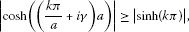(3.9)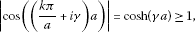(3.10)

which hold for all $k\in \mathbb{Z}$ with $|k|\ge {\stackrel{ˆ}{k}}_{0}$ and all $\gamma \in \mathbb{R}$. Therefore, it follows from (3.6), (3.8)-(3.10) and the corresponding estimates with μ replaced by that there is ${\stackrel{ˆ}{k}}_{1}$ such that $|{\varphi }_{1}\left(\mu \right)|<1$ for all $\mu \in {S}_{k}$ with $k>{\stackrel{ˆ}{k}}_{1}$. Again from the definition of ${\varphi }_{1}$ in (3.4) and Rouché’s theorem, we conclude that the functions ${\varphi }_{0}$ and ϕ have the same number of zeros in the square ${S}_{k}$, for $k\in \mathbb{N}$ with $k\ge {\stackrel{ˆ}{k}}_{1}$.

Since ${\varphi }_{0}$ has $4k+2$ zeros inside ${S}_{k}$ and thus $4k+2+4$ zeros inside ${S}_{k+1}$, it follows that ϕ has no large zeros other than the zeros $±{\stackrel{ˆ}{\mu }}_{k}$ found above for $|k|$ sufficiently large, and that ${\stackrel{ˆ}{\lambda }}_{k}={\stackrel{ˆ}{\mu }}_{k}^{2}$ account for all eigenvalues of the problem (2.1)-(2.2) since each of these eigenvalues gives rise to two zeros of ϕ, counted with multiplicity. By Proposition 2.3, all eigenvalues with non-zero real part occur in pairs ${\stackrel{ˆ}{\lambda }}_{k}$, $-\overline{{\stackrel{ˆ}{\lambda }}_{k}}$, which shows that we can index all such eigenvalues as ${\stackrel{ˆ}{\lambda }}_{-k}=-\overline{{\stackrel{ˆ}{\lambda }}_{k}}$. Since there is an odd number of remaining indices, the number of pure imaginary eigenvalues must be odd.

Case 2: The function ϕ in this case is

$\begin{array}{rcl}\varphi \left(\mu \right)& =& -\frac{1}{2}\left(2{\alpha }^{2}+1\right)\mu \left[cosh\left(\mu a\right)sin\left(\mu a\right)-sinh\left(\mu a\right)cos\left(\mu a\right)\right]\\ -\frac{1}{2}{\alpha }^{2}{\mu }^{3}\left[sinh\left(\mu a\right)cos\left(\mu a\right)+cosh\left(\mu a\right)sin\left(\mu a\right)\right]\\ +\frac{1}{2}i\alpha {\mu }^{2}\left[3cosh\left(\mu a\right)cos\left(\mu a\right)+1\right]+\frac{1}{2}i{a}^{3}{\mu }^{2}\left[cosh\left(\mu a\right)cos\left(\mu a\right)-1\right]\\ +i\alpha {\mu }^{2}sin\left(\mu a\right)sinh\left(\mu a\right).\end{array}$

Then all the estimates are as in Case 4, and the result in Case 2 immediately follows from that in Case 4 if we observe that each ${S}_{k}$ for k large enough contains two fewer zeros of ϕ than in Case 4.

Case 1: A straightforward calculation gives

$\begin{array}{rcl}\varphi \left(\mu \right)& =& {\alpha }^{2}{\mu }^{6}sin\left(\mu a\right)sinh\left(\mu a\right)-\frac{1}{2}\left(1+3{\alpha }^{2}\right){\mu }^{4}cos\left(\mu a\right)cosh\left(\mu a\right)\\ -\frac{1}{2}i\left(2\alpha +{\alpha }^{3}\right){\mu }^{5}\left(sin\left(\mu a\right)cosh\left(\mu a\right)+cos\left(\mu a\right)sinh\left(\mu a\right)\right)\\ -\frac{1}{2}i\alpha {\mu }^{3}\left(sin\left(\mu a\right)cosh\left(\mu a\right)-cos\left(\mu a\right)sinh\left(\mu a\right)\right)+\frac{1}{2}\left(1-{\alpha }^{2}\right){\mu }^{4}.\end{array}$

Then

$\begin{array}{rcl}{\varphi }_{1}\left(\mu \right)& =& \frac{2\varphi \left(\mu \right)+{\alpha }^{2}{\varphi }_{0}\left(\mu \right)}{{\varphi }_{0}\left(\mu \right)}\\ =& \frac{1+3{\alpha }^{2}}{2{\mu }^{2}}cot\left(\mu a\right)coth\left(\mu a\right)+\frac{\left(2\alpha +{\alpha }^{3}\right)i}{2\mu }\left[coth\left(\mu a\right)+cot\left(\mu a\right)\right]\\ +\frac{i\alpha }{2{\mu }^{3}}\left[coth\left(\mu a\right)-cot\left(\mu a\right)\right]-\frac{1-{\alpha }^{2}}{2{\mu }^{2}}\frac{1}{sin\left(\mu a\right)sinh\left(\mu a\right)}.\end{array}$

The result follows with reasonings and estimates as in the proof of Case 4, replacing μ by $\mu ±\frac{\pi }{2}$ and $\mu ±i\frac{\pi }{2}$, respectively.

Case 3: The function ϕ in this case is

$\begin{array}{rcl}\varphi \left(\mu \right)& =& -i\alpha {\mu }^{4}sin\left(\mu a\right)sinh\left(\mu a\right)+\frac{1}{2}i\left(3\alpha +{\alpha }^{3}\right){\mu }^{2}cos\left(\mu a\right)cosh\left(\mu a\right)\\ -\frac{1}{2}\left(2{\alpha }^{2}+1\right){\mu }^{3}\left(sin\left(\mu a\right)cosh\left(\mu a\right)+cos\left(\mu a\right)sinh\left(\mu a\right)\right)\\ -\frac{1}{2}{\alpha }^{2}\mu \left(sin\left(\mu a\right)cosh\left(\mu a\right)-cos\left(\mu a\right)sinh\left(\mu a\right)\right)+\frac{1}{2}i\left(\alpha -{\alpha }^{3}\right){\mu }^{2},\end{array}$

and a reasoning as in Case 1 completes the proof. □

## 4 Birkhoff regularity

We refer to [, Definition 7.3.1] for the definition of the Birkhoff regularity.

Proposition 4.1 The boundary value problem (2.1), (2.5)-(2.8) is Birkhoff regular for $\alpha >0$ with respect to the eigenvalue parameter μ given by $\lambda ={\mu }^{2}$.

Proof The characteristic function of (2.1) as defined in [, (7.1.4)] is $\pi \left(\rho \right)={\rho }^{4}-1$, and its zeros are ${i}^{k-1}$, $k=1,\dots ,4$. We can choose

$C\left(x,\mu \right)=diag\left(1,\mu ,{\mu }^{2},{\mu }^{3}\right){\left({i}^{\left(k-1\right)\left(j-1\right)}\right)}_{k,j=1}^{4}$

according to [, Theorem 7.2.4.A]. The boundary condition (2.5)-(2.8) can be written in the form

${B}_{j}\left(\lambda \right)y={\stackrel{ˆ}{B}}_{j}\left(\mu \right)\left(y\left({a}_{j}\right),{y}^{\prime }\left({a}_{j}\right),{y}^{″}\left({a}_{j}\right),{y}^{\left(3\right)}\left({a}_{j}\right)\right),\phantom{\rule{1em}{0ex}}j=1,2,3,4,$

where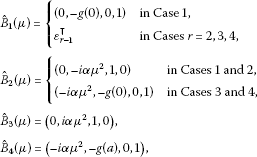and where ${\epsilon }_{\nu }$ denotes the ν th unit vector in ${\mathbb{C}}^{4}$. Thus the boundary matrices defined in [, (7.3.1)] are given by

${W}^{\left(0\right)}\left(\mu \right)=\left(\begin{array}{c}{\stackrel{ˆ}{B}}_{1}\\ {\stackrel{ˆ}{B}}_{2}\left(\mu \right)\\ 0\\ 0\end{array}\right)C\left(0,\mu \right),\phantom{\rule{2em}{0ex}}{W}^{\left(1\right)}\left(\mu \right)=\left(\begin{array}{c}0\\ 0\\ {\stackrel{ˆ}{B}}_{3}\left(\mu \right)\\ {\stackrel{ˆ}{B}}_{4}\left(\mu \right)\end{array}\right)C\left(a,\mu \right).$

Choosing ${C}_{2}\left(\mu \right)=diag\left({\mu }^{{p}_{1}},{\mu }^{3},{\mu }^{3},{\mu }^{3}\right)$, it follows that ${C}_{2}{\left(\mu \right)}^{-1}{W}^{\left(j\right)}\left(\mu \right)={W}_{0}^{\left(j\right)}+O\left({\mu }^{-1}\right)$, where

${W}_{0}^{\left(0\right)}=\left(\begin{array}{cccc}1& {i}^{r-2}& {i}^{2\left(r-2\right)}& {i}^{3\left(r-2\right)}\\ {\theta }_{1}& {\theta }_{2}& {\theta }_{3}& {\theta }_{4}\\ 0& 0& 0& 0\\ 0& 0& 0& 0\end{array}\right),\phantom{\rule{2em}{0ex}}{W}_{0}^{\left(1\right)}=\left(\begin{array}{cccc}0& 0& 0& 0\\ 0& 0& 0& 0\\ i\alpha & -\alpha & -i\alpha & \alpha \\ 1& -i& -1& i\end{array}\right),$

for Case r and ${\theta }_{j}=-{i}^{j}\alpha$ for Cases 1 and 2, while ${\theta }_{j}={\left(-i\right)}^{j-1}$ for Cases 3 and 4. The Birkhoff matrices are

${W}_{0}^{\left(0\right)}{\mathrm{\Delta }}_{j}+{W}_{0}^{\left(1\right)}\left(I-{\mathrm{\Delta }}_{j}\right),$
(4.1)

where ${\mathrm{\Delta }}_{j}$, $j=1,2,3,4$ are the $4×4$ diagonal matrices with 2 consecutive ones and 2 consecutive zeros in the diagonal in a cyclic arrangement, see [, Definition 7.3.1 and Proposition 4.1.7]. It is easy to see that after a permutation of columns, the matrices (4.1) are block diagonal matrices consisting of $2×2$ blocks taken from two consecutive columns (in the sense of cyclic arrangement) of the first two rows of ${W}_{0}^{\left(0\right)}$ and the last two rows of ${W}_{0}^{\left(1\right)}$, respectively. Hence the determinants of the Birkhoff matrices (4.1) are

$±|\begin{array}{cc}{i}^{\left(j-1\right)\left(r-2\right)}& {i}^{j\left(r-2\right)}\\ -{i}^{j}\alpha & -{i}^{j+1}\alpha \end{array}||\begin{array}{cc}{i}^{j+2}\alpha & {i}^{j+3}\alpha \\ {\left(-i\right)}^{j+1}& {\left(-i\right)}^{j+2}\end{array}|=±{i}^{j\left(r-1\right)}\left(1+{i}^{1-r}\right)2{\alpha }^{2}\ne 0$

in Cases 1 and 2, i.e., $r\in \left\{1,2\right\}$, whereas

$±|\begin{array}{cc}{i}^{\left(j-1\right)\left(r-2\right)}& {i}^{j\left(r-2\right)}\\ {\left(-i\right)}^{\left(j-1\right)}& {\left(-i\right)}^{j}\end{array}||\begin{array}{cc}{i}^{j+2}\alpha & {i}^{j+3}\alpha \\ {\left(-i\right)}^{j+1}& {\left(-i\right)}^{j+2}\end{array}|=±{i}^{j\left(r-3\right)}\left({i}^{2-r}-i\right)2\alpha \ne 0,$

in Cases 3 and 4. Thus, the problem (2.1), (2.5)-(2.8) is Birkhoff regular. □

## 5 Asymptotic expansions of eigenvalues

Let D, as a function of μ with $\lambda ={\mu }^{2}$, be the characteristic function of the problem (2.1), (2.5)-(2.8) with respect to the fundamental system ${y}_{j}$, $j=1,2,3,4$, with ${y}_{j}^{\left[m\right]}\left(0\right)={\delta }_{j,m+1}$ for $m=0,1,2,3$, where δ is the Kronecker delta. Denote by ${D}_{0}$ the corresponding characteristic function for $g=0$. Note that the characteristic functions ${D}_{0}$ and ${\varphi }_{0}$ considered in Section 3 have the same zeros counted with multiplicity. Due to the Birkhoff regularity, g only influences lower order terms in D. Therefore, it can be inferred that outside the interior of the small squares ${R}_{k}$, $-{R}_{k}$, $i{R}_{k}$, $-i{R}_{-k}$ around the zeros of ${D}_{0}$, $|D\left(\mu \right)-{D}_{0}\left(\mu \right)|<|{D}_{0}\left(\mu \right)|$ if $|\mu |$ is sufficiently large. Since the fundamental system ${y}_{j}$, $j=1,2,3,4$, depends analytically on μ, also D and ${D}_{0}$ are analytic functions. Hence, applying Rouché’s theorem both to the large squares ${S}_{k}$ and to the small squares which are sufficiently far away from the origin, it follows that the eigenvalues of the boundary value problem for general g have the same asymptotic distribution as for $g=0$. Whence Proposition 3.2 leads to

Proposition 5.1 For $g\in {C}^{1}\left[0,a\right]$, there exists a positive integer ${k}_{0}$ such that the eigenvalues ${\stackrel{ˆ}{\lambda }}_{k}$, counted with multiplicity, of the problem (2.1), (2.5)-(2.8), where $k\in \mathbb{Z}\setminus \left\{0\right\}$ in Cases  1 and  2 and $k\in \mathbb{Z}$ in Cases 3 and 4, can be enumerated in such a way that the eigenvalues ${\lambda }_{k}$ are pure imaginary for $|k|<{k}_{0}$, and ${\lambda }_{-k}=-\overline{{\lambda }_{k}}$ for $k\ge {k}_{0}$. For $k>0$, we can write ${\lambda }_{k}={\mu }_{k}^{2}$, where the ${\mu }_{k}$ have the following asymptotic representation as $k\to \mathrm{\infty }$: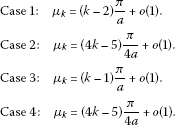In particular, the number of pure imaginary eigenvalues is even in Cases 1 and 2 and odd in Cases 3 and 4.

In the remainder of the section, we are going to establish more precise asymptotic expansions of the eigenvalues. According to [, Theorem 8.2.1], (2.1) has an asymptotic fundamental system $\left\{{\eta }_{1},{\eta }_{2},{\eta }_{3},{\eta }_{4}\right\}$ of the form

${\eta }_{\nu }^{\left(j\right)}\left(x,\mu \right)={\delta }_{\nu ,j}\left(x,\mu \right){e}^{{i}^{\nu -1}\mu x};\phantom{\rule{1em}{0ex}}\nu =1,\dots ,4;j=0,\dots ,3;$
(5.1)

where

${\delta }_{\nu ,j}\left(x,\mu \right)=\left[\frac{{d}^{j}}{d{x}^{j}}\right]\left\{\sum _{r=0}^{4}{\left(\mu {i}^{\nu -1}\right)}^{-r}{\phi }_{r}\left(x\right){e}^{{i}^{\nu -1}\mu x}\right\}{e}^{-{i}^{\nu -1}\mu x}+o\left({\mu }^{-4+j}\right),$
(5.2)

and $\left[\frac{{d}^{j}}{d{x}^{j}}\right]$ means that we omit those terms of the Leibniz expansion which contain a function ${\phi }_{r}^{\left(k\right)}$ with $k>4-r$. Since the coefficient of ${y}^{\left(3\right)}$ in (2.1) is zero, we have ${\phi }_{0}\left(x\right)=1$, see [, (8.2.3)].

We will now determine the functions ${\phi }_{1}$ and ${\phi }_{2}$. In this regard, observe that ${n}_{0}=0$ and $l=4$ in the notation of [, (8.1.2) and (8.1.3)], see [, Theorem 8.1.2]. From [, (8.2.45)], we know that

${\phi }_{r}={\phi }_{1,r}={\epsilon }_{1}^{\mathsf{T}}V{Q}^{\left[r\right]}{\epsilon }_{1},$
(5.3)

where ${\epsilon }_{\nu }$ is the ν th unit vector in ${\mathbb{C}}^{4}$, $V={\left({i}^{\left(j-1\right)\left(k-1\right)}\right)}_{j,k=1}^{4}$, and ${Q}^{\left[r\right]}$ are $4×4$ matrices given by [, (8.2.28), (8.2.33) and (8.2.34)], that is, ${Q}^{\left[0\right]}={I}_{4}$,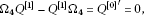(5.4)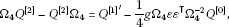(5.5)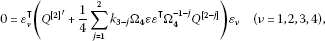(5.6)

where ${k}_{2}=-g$, ${k}_{1}=-{g}^{\prime }$, ${\mathrm{\Omega }}_{4}=diag\left(1,i,-1,-i\right)$ and ${\epsilon }^{\mathsf{T}}=\left(1,1,1,1\right)$. Let $G\left(x\right)={\int }_{0}^{x}g\left(t\right)\phantom{\rule{0.2em}{0ex}}dt$. A lengthy but straightforward calculation gives

${\phi }_{1}=\frac{1}{4}G,\phantom{\rule{2em}{0ex}}{\phi }_{2}=\frac{1}{32}{G}^{2}-\frac{1}{8}g,$
(5.7)

and thus

$\begin{array}{rcl}{\eta }_{\nu }\left(x,\mu \right)& =& \left(1+\frac{1}{4}{i}^{-\nu +1}G\left(x\right){\mu }^{-1}+{\left(-1\right)}^{\nu -1}\left(\frac{1}{32}G{\left(x\right)}^{2}-\frac{1}{8}g\left(x\right)\right){\mu }^{-2}\right){e}^{{i}^{\nu -1}\mu x}\\ +{\left\{o\left({\mu }^{-2}\right)\right\}}_{\mathrm{\infty }}{e}^{{i}^{\nu -1}\mu x}\end{array}$
(5.8)

for $\nu =1,2,3,4$, where ${\left\{o\left(\cdot \right)\right\}}_{\mathrm{\infty }}$ means that the estimate is uniform in x.

The characteristic function of (2.1), (2.5)-(2.8) is

$D\left(\mu \right)=det{\left({\gamma }_{jk}exp\left({\epsilon }_{jk}\right)\right)}_{j,k=1}^{4},$

where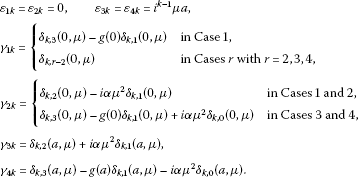Note that

$D\left(\mu \right)=\sum _{m=1}^{5}{\psi }_{m}\left(\mu \right){e}^{{\omega }_{m}\mu a},$
(5.9)

where ${\omega }_{1}=1+i$, ${\omega }_{2}=-1+i$, ${\omega }_{3}=-1-i$, ${\omega }_{4}=1-i$, ${\omega }_{5}=0$, and each of the functions ${\psi }_{1},\dots ,{\psi }_{5}$ has asymptotic representations of the form ${c}_{k}{\mu }^{k}+{c}_{k-1}{\mu }^{k-1}+\dots +{c}_{{k}_{0}}{\mu }^{{k}_{0}}+o\left({\mu }^{{k}_{0}}\right)$.

It follows from (5.9) that

${D}_{1}\left(\mu \right):=D\left(\mu \right){e}^{-{\omega }_{1}\mu a}={\psi }_{1}\left(\mu \right)+\sum _{m=2}^{5}{\psi }_{m}\left(\mu \right){e}^{\left({\omega }_{m}-{\omega }_{1}\right)\mu a},$
(5.10)

where ${\omega }_{2}-{\omega }_{1}=-2$, ${\omega }_{3}-{\omega }_{1}=-2-2i$, ${\omega }_{4}-{\omega }_{1}=-2i$, ${\omega }_{5}-{\omega }_{1}=-1-i$. If $arg\mu \in \left[-\frac{3\pi }{8},\frac{\pi }{8}\right]$, we have $|{e}^{\left({\omega }_{m}-{\omega }_{1}\right)\mu a}|\le {e}^{-sin\frac{\pi }{8}|\mu |a}$ for $m=2,3,5$ and the terms ${\psi }_{m}\left(\mu \right){e}^{\left({\omega }_{m}-{\omega }_{1}\right)\mu a}$ for $m=2,3,5$ can be absorbed by ${\psi }_{1}\left(\mu \right)$ as they are of the form $o\left({\mu }^{-s}\right)$ for any integer s. Hence, for $arg\mu \in \left[-\frac{3\pi }{8},\frac{\pi }{8}\right]$,

${D}_{1}\left(\mu \right)={\psi }_{1}\left(\mu \right)+{\psi }_{4}\left(\mu \right){e}^{\left({\omega }_{4}-{\omega }_{3}\right)\mu a}={\psi }_{1}\left(\mu \right)+{\psi }_{4}\left(\mu \right){e}^{-2i\mu a},$
(5.11)

where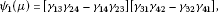(5.12)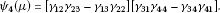(5.13)

A straightforward calculation gives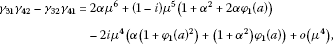(5.14)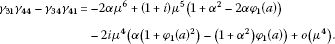(5.15)

For the other two factors in (5.12) and (5.13), we have to consider the four different cases.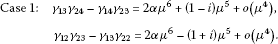Therefore,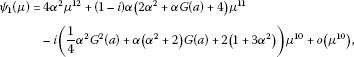(5.16)(5.17)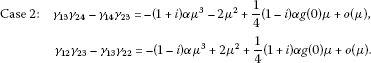Thus, we have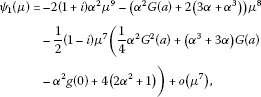(5.18)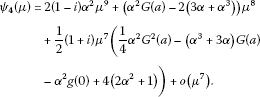(5.19)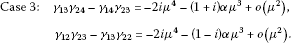Hence, we get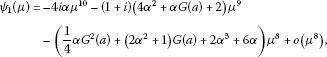(5.20)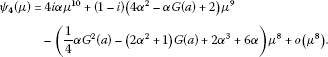(5.21)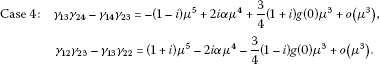Thus, we have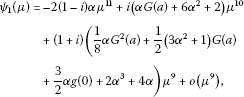(5.22)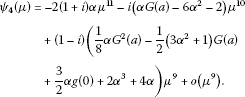(5.23)

We already know by Proposition 5.1 that the zeros ${\mu }_{k}$ of D satisfy the asymptotic representations ${\mu }_{k}=k\frac{\pi }{a}+{\tau }_{0}+o\left(1\right)$ as $k\to \mathrm{\infty }$. In order to improve on these asymptotic representations, write

${\mu }_{k}=k\frac{\pi }{a}+\tau \left(k\right),\phantom{\rule{1em}{0ex}}\tau \left(k\right)=\sum _{m=0}^{n}{\tau }_{m}{k}^{-m}+o\left({k}^{-n}\right),k=1,2,\dots .$
(5.24)

Because of the symmetry of the eigenvalues, we will only need to find the asymptotic expansions as $k\to \mathrm{\infty }$. We know ${\tau }_{0}$ from Proposition 5.1, and it is our aim to find ${\tau }_{1}$ and ${\tau }_{2}$. To this end, we will substitute (5.24) into ${D}_{1}\left({\mu }_{k}\right)=0$ and we will then compare the coefficients of ${k}^{0}$, ${k}^{-1}$ and ${k}^{-2}$.

Observe that

$\begin{array}{rl}{e}^{-2i{\mu }_{k}a}& ={e}^{-2i\tau \left(k\right)a}={e}^{-2i{\tau }_{0}a}exp\left(-2ia\left(\frac{{\tau }_{1}}{k}+\frac{{\tau }_{2}}{{k}^{2}}+o\left({k}^{-2}\right)\right)\right)\\ ={e}^{-2i{\tau }_{0}a}\left(1-2ia{\tau }_{1}\frac{1}{k}-\left(2{a}^{2}{\tau }_{1}^{2}+2ia{\tau }_{2}\right)\frac{1}{{k}^{2}}+o\left({k}^{-2}\right)\right),\end{array}$
(5.25)

while

$\frac{1}{{\mu }_{k}}=\frac{a}{\pi k}{\left(1+\frac{a\tau \left(k\right)}{k\pi }\right)}^{-1}=\frac{a}{k\pi }-\frac{{a}^{2}{\tau }_{0}}{{k}^{2}{\pi }^{2}}+o\left({k}^{-2}\right).$
(5.26)

Using (5.11), ${D}_{1}\left({\mu }_{k}\right)=0$ can be written as

${\mu }_{k}^{-\gamma }{\psi }_{1}\left({\mu }_{k}\right)+{\mu }_{k}^{-\gamma }{\psi }_{4}\left({\mu }_{k}\right){e}^{-2i{\tau }_{k}a}=0,$
(5.27)

where γ is the highest μ-power in ${\psi }_{1}\left(\mu \right)$ and ${\psi }_{4}\left(\mu \right)$. Substituting (5.25) and (5.26) into (5.27) and comparing the coefficients of ${k}^{0}$, ${k}^{-1}$ and ${k}^{-2}$, we get

Theorem 5.2 For $g\in {C}^{1}\left[0,a\right]$, there exists a positive integer ${k}_{0}$ such that the eigenvalues ${\lambda }_{k}$, $k\in \mathbb{Z}$, counted with multiplicity, of the problem (2.1), (2.5)-(2.8), where $k\in \mathbb{Z}\setminus \left\{0\right\}$ in Cases  1 and 2 and $k\in \mathbb{Z}$ in Cases 3 and 4, can be enumerated in such a way that the eigenvalues ${\lambda }_{k}$ are pure imaginary for $|k|<{k}_{0}$, and ${\lambda }_{-k}=-\overline{{\lambda }_{k}}$ for $k\ge {k}_{0}$, where ${\lambda }_{k}={\mu }_{k}^{2}$ and the ${\mu }_{k}$ have the asymptotic representations

${\mu }_{k}=k\frac{\pi }{a}+{\tau }_{0}+\frac{{\tau }_{1}}{k}+\frac{{\tau }_{2}}{{k}^{2}}+o\left({k}^{-2}\right)$

and the numbers ${\tau }_{0}$, ${\tau }_{1}$, ${\tau }_{2}$ are as follows: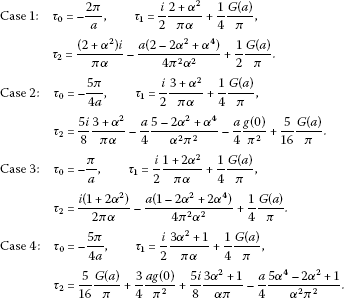In particular, the number of pure imaginary eigenvalues is even in Cases 1 and 2 and odd in Cases 3 and 4.

Remark 5.3 In  we have considered the differential equation (2.1) with the same boundary conditions ${B}_{3}$, ${B}_{4}$ at a as in this paper but with λ-independent boundary conditions at 0, that is, the boundary conditions ${B}_{1}$ also occur in . Whereas in  the number of pure imaginary eigenvalues is odd in each case, this number is even in Cases 1 and 2 of this paper. We observe that in Cases 1 and 2, the λ-dependent part is the ‘dominating’ part of the boundary condition ${B}_{2}$, in the sense that it has the highest μ-power arising as ${\mu }^{2j+k}$ from ${\lambda }^{j}{y}^{\left[k\right]}$, whereas in Cases 3 and 4 the λ-independent part is dominating. It may be interesting to investigate if, in general, the parity of the number of pure imaginary eigenvalues can be determined by the number of dominating λ-dependent parts in the boundary conditions.

We can observe that the functions ${\varphi }_{0}$ in the Cases 3 and 4 are respectively the same as in  since the corresponding dominating terms in the boundary conditions coincide. However, the numbers ${\tau }_{1}$ and ${\tau }_{2}$ differ from those of  in each case, which is due to the λ-term in the boundary condition ${B}_{2}$.

## References

1. Kerimov NB, Aliev ZS: Basis properties of a spectral problem with a spectral parameter in the boundary condition (Russian). Mat. Sb. 2006, 197(10):65-86. translation in Sb. Math. 197, 1467-1487 (2006) 10.4213/sm1433

2. Kerimov NB, Aliev ZS: On the basis property of the system of eigenfunctions of a spectral problem with a spectral parameter in the boundary condition (Russian). Differ. Uravn. 2007, 43: 886-895. translation in Differ. Equ. 43, 905-915 (2007)

3. Marletta M, Shkalikov A, Tretter C: Pencils of differential operators containing the eigenvalue parameter in the boundary conditions. Proc. R. Soc. Edinb., Sect. A, Math. 2003, 133: 893-917. 10.1017/S0308210500002730

4. Shkalikov AA: Boundary problems for ordinary differential equations with parameter in the boundary conditions (Russian). Tr. Semin. Im. I.G. Petrovskogo 1983, 9: 190-229. translation in J. Sov. Math. 33, 1311-1342 (1986)

5. Wang A, Sun J, Zettl A: The classification of self-adjoint boundary conditions: separated, coupled, and mixed. J. Funct. Anal. 2008, 255: 1554-1573. 10.1016/j.jfa.2008.05.003

6. Wang A, Sun J, Zettl A: Characterization of domains of self-adjoint ordinary differential operators. J. Differ. Equ. 2009, 246: 1600-1622. 10.1016/j.jde.2008.11.001

7. Möller M, Zettl A: Symmetric differential operators and their Friedrichs extension. J. Differ. Equ. 1995, 115: 50-69. 10.1006/jdeq.1995.1003

8. Weidmann J Lect. Notes Math. 1258. In Spectral Theory of Ordinary Differential Operators. Springer, Berlin; 1987.

9. Behncke H: Spectral analysis of fourth order differential operators. I. Math. Nachr. 2006, 279: 58-72. 10.1002/mana.200310345

10. Behncke H: Spectral analysis of fourth order differential operators. II. Math. Nachr. 2006, 279: 73-85. 10.1002/mana.200310346

11. Pivovarchik V, van der Mee C: The inverse generalized Regge problem. Inverse Probl. 2001, 17: 1831-1845. 10.1088/0266-5611/17/6/318

12. Möller M, Pivovarchik V: Spectral properties of a fourth order differential equation. J. Anal. Appl. 2006, 25: 341-366.

13. Möller M, Zinsou B: Self-adjoint fourth order differential operators with eigenvalue parameter dependent boundary conditions. Quaest. Math. 2011, 34: 393-406. doi:10.2989/16073606.2011.622913 10.2989/16073606.2011.622913

14. Möller M, Zinsou B: Spectral asymptotics of self-adjoint fourth order differential operators with eigenvalue parameter dependent boundary conditions. Complex Anal. Oper. Theory 2012, 6: 799-818. doi:10.1007/s11785-011-0162-1 10.1007/s11785-011-0162-1

15. Mennicken R, Möller M North-Holland Mathematics Studies 192. In Non-self Adjoint Boundary Eigenvalue Problems. Elsevier, Amsterdam; 2003.

## Acknowledgements

This research was partially supported by a grant from the NRF of South Africa, Grant number 69659. Various of the above calculations have been verified with Sage.

## Author information

Authors

### Corresponding author

Correspondence to Manfred Möller.

### Competing interests

The authors do not have any competing interests.

### Authors’ contributions

The subject of this paper is part of the PhD thesis of BZ. The subject has been suggested and supervised by MM, and the initial version of the paper has been written by BZ. The submitted version has been verified and discussed by MM and BZ.

## Rights and permissions

Reprints and Permissions

Möller, M., Zinsou, B. Spectral asymptotics of self-adjoint fourth order boundary value problems with eigenvalue parameter dependent boundary conditions. Bound Value Probl 2012, 106 (2012). https://doi.org/10.1186/1687-2770-2012-106

• Accepted:

• Published:

• DOI: https://doi.org/10.1186/1687-2770-2012-106

### Keywords

• fourth order boundary value problems